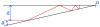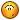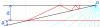BrainDen.com - Brain Teasers
• 0

# The "aha!" problems 8: Reflect on this

## Question

A ray of light encounters a pair of angled mirrors from the left, as shown, a distance d from one mirror and at an angle a from the other mirror. If angle a is exactly 22.5o, how close will the ray get to their intersection at point O before it eventually exits again to the left? The drawing may not be to scale.•1

## Recommended Posts

• 0

When the light starts to come back out it is d units from the intersection along the top line. And as long is my thought process is correct the light will follow the exact same path in and out of the mirrors.

##### Share on other sites

• 0

When the light starts to come back out it is d units from the intersection along the top line. And as long is my thought process is correct the light will follow the exact same path in and out of the mirrors.

Wow ... talk about a "bait-and-switch." And this is such a nice analysis ...

Our Puzzle Quality Control Department has informed us that we cannot

claim an "aha!" status for a puzzle that admits to a straightforward constructive

solution, as this one does, brilliant and insightful though it may be.

They inform us that it was incorrect on our part to specify the angle a at all.

Ouch. We do apologize.Can the puzzle still be answered?

##### Share on other sites

• 0

By the way I am marking Rob_G's answer as the solution.

Still, for a coveted bonanova Gold Star, can RG's answer be shown to be completely independent of a?

It's a pretty proof.

##### Share on other sites

• 0

Lets see what the light ray sees. The light ray, is travelling straight parallel to the horizontal mirror. It encounters a plane inclined at angle a. We can assume it keeps travelling straight through without getting reflected, and then it meets another surface inclined at 2a. it keeps travelling straight through, without getting reflected and meets the third surface at 3a, and so on.

It will be similar, if all these surfaces are assumed to be originating from O, inclined at a, 2a, 3a, .. angles in anticlockwise direction, and the light ray keeps going straight, cutting through all these surfaces. The light ray, thus, starts exiting from left, when it hits the first surface which is inclined at 90 degrees or more to the horizontal.

If 'a' is a factor of 90 degree, it will cut this surface exactly at d distance.

e.g, if a=30:

imagine three imaginary surfaces, inclined at 30, 60 and 90, all intersecting at O. Imagine the ray travelling horizontally, d distance below the horizontal mirror, cutting through these three surfaces and finally hitting the 90 degree mirror at d distance below O. Hence, it exits exactly at d distance from O IF a is a factor of 90.

but, if a is not a factor of 90 (but less than 90), then the distance is d/cos(ma mod 90) where ma>90>(m-1)a

In general, for any a, distance = d/cos(ma mod 90) where ma>=90>(m-1)a

##### Share on other sites

• 0

Lets see what the light ray sees. The light ray, is travelling straight parallel to the horizontal mirror. It encounters a plane inclined at angle a. We can assume it keeps travelling straight through without getting reflected, and then it meets another surface inclined at 2a. it keeps travelling straight through, without getting reflected and meets the third surface at 3a, and so on.

It will be similar, if all these surfaces are assumed to be originating from O, inclined at a, 2a, 3a, .. angles in anticlockwise direction, and the light ray keeps going straight, cutting through all these surfaces. The light ray, thus, starts exiting from left, when it hits the first surface which is inclined at 90 degrees or more to the horizontal.

If 'a' is a factor of 90 degree, it will cut this surface exactly at d distance.

e.g, if a=30:

imagine three imaginary surfaces, inclined at 30, 60 and 90, all intersecting at O. Imagine the ray travelling horizontally, d distance below the horizontal mirror, cutting through these three surfaces and finally hitting the 90 degree mirror at d distance below O. Hence, it exits exactly at d distance from O IF a is a factor of 90.

but, if a is not a factor of 90 (but less than 90), then the distance is d/cos(ma mod 90) where ma>90>(m-1)a

In general, for any a, distance = d/cos(ma mod 90) where ma>=90>(m-1)a

You have it for submultiples of pi radians.

Can we generalize to arbitrary a?

##### Share on other sites

• 0

hi bonanova,

i have given it for any arbitrary a (both when its sub multiple and when its not).

only case to be added is, if a is integer multiple of pi, then the ray doesnt return at all

##### Share on other sites

• 0

Maybe I misunderstood. You may have the answer without actually saying it.

You're certainly on the right track. The question what is the distance of closest approach to O,

and there is no condition that it must coincide with the incident ray when it leaves.

##### Share on other sites

• 0

Yes, bonanova. I think the answer depends on a (e.g. if a is obtuse the ray doesnt exit on left and never hits the top mirror). It coinicides with the icident ray if a is a factor of pi/2 radian

##### Share on other sites

• 0

Yes, bonanova. I think the answer depends on a (e.g. if a is obtuse the ray doesnt exit on left and never hits the top mirror). It coinicides with the icident ray if a is a factor of pi/2 radian

Everything in your post is correct. But remember what the question is.

Whatever value a has, how close does the ray come to the point O?

The phrase "before it exits to the left" is appropriate to a being acute, as in the figure.

If you want to include a being obtuse, so that the ray would not exit to the left,

that just gives you "forever" as the time frame to consider the ray's point of closest approach.

Although of course you wouldn't need "forever" to determine it.

In other words, the wording is not meant to be tricky: assume a to be acute if you like.

##### Share on other sites

• 0

Given angle a find ceiling(90/a)=n a*n will be the angle made where the light will start to come backout. The distance from the intersection should be d/sin(n*a)

Edited by Rob_G
•1
##### Share on other sites

• 0

Yes, bonanova. I think the answer depends on a (e.g. if a is obtuse the ray doesnt exit on left and never hits the top mirror). It coinicides with the icident ray if a is a factor of pi/2 radian

Everything in your post is correct. But remember what the question is.

Whatever value a has, how close does the ray come to the point O?

The phrase "before it exits to the left" is appropriate to a being acute, as in the figure.

If you want to include a being obtuse, so that the ray would not exit to the left,

that just gives you "forever" as the time frame to consider the ray's point of closest approach.

Although of course you wouldn't need "forever" to determine it.

In other words, the wording is not meant to be tricky: assume a to be acute if you like.

Hi Bonanova,

Sorry, I do not understand. Is my answer wrong? or incomplete?

##### Share on other sites

• 0

I probably muddied the waters by at first specifying a to be 22.5o. My bad.

It got people to worry about the exiting trajectory of the ray, a matter that is irrelevant to the question asked.

The Aha! question asks (only) for the distance from the ray to the point
O at its point of closest approach. The Aha! moment comes with the realization that this distance has no dependence whatsoever on the value of a.

If a is obtuse, the ray remains a distance d below the horizontal mirror until after its closest approach.

It strikes the angled mirror after it has passed the point O.

That leaves the acute case. And here we do a little trick. On each occasion that the ray reflects from a mirror, we rotate the mirrors about an axis formed by O and the point of reflection. At each rotation the mirrors take the successive positions shown in light blue. Because the axis of rotation in each case contains the point O, the rotation does not change the distance between O and the ray. That justifies the drawing shown, where the ray can be assumed to continue as if not reflected at all. The ray approaches the point O along the path parallel to and a distance d below the upper mirror's original position, and therefore also a distance d below the point O.

This result and the implications of the drawing do not depend in any way on the value of a.

A final point of clarification is that we are not seeking the point of reflection that is closest to O. In general the point of closest approach will occur between reflections. Another reason that initially saying a was 22.5o may have been confusing.##### Share on other sites

• 0

understood the exact question now## Join the conversation

You can post now and register later. If you have an account, sign in now to post with your account.×   Pasted as rich text.   Paste as plain text instead

Only 75 emoji are allowed.

×   Your previous content has been restored.   Clear editor

×   You cannot paste images directly. Upload or insert images from URL.